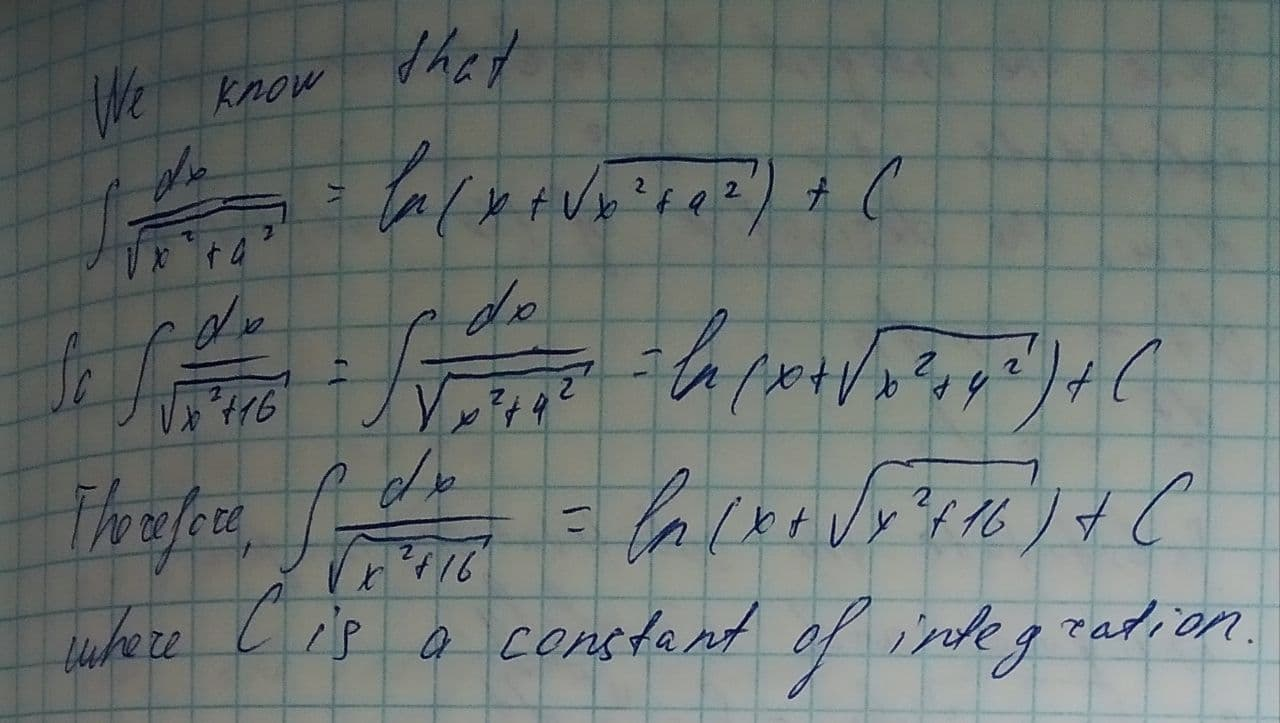# Use a table of integrals to evaluate the following indefinite integrals \int \frac{dx}{\sqrt{x^{2}+16}}Ramsey 2021-08-12 Answered
Use a table of integrals to evaluate the following indefinite integrals.
$$\displaystyle\int{\frac{{{\left.{d}{x}\right.}}}{{\sqrt{{{x}^{{{2}}}+{16}}}}}}$$

• Questions are typically answered in as fast as 30 minutes

### Plainmath recommends

• Get a detailed answer even on the hardest topics.
• Ask an expert for a step-by-step guidance to learn to do it yourself.2k1enyvp
To evaluate: